• Problem Description ZZZ is an enthusiastic ACMer and he spends lots of time on training. He always stays up late for training. He needs enough time to sleep, and hates skipping classes....Golang erlang r语言
• Problem Description Beggar My Neighbour'' (sometimes known as Strip Jack Naked'') is a traditional card game, designed to help teach beginners something about cards and their values....Golang erlang r语言
• 题目描述 分析 ...一种很直接的方法便是等于左子树的结点数+右子树的节点数+1。至此，我们就大概知道代码怎么写了。如果刚好是满二叉树，那么节点数便是2^h-1;如果不是，那么就左子树、右子树的结点数分别加
题目描述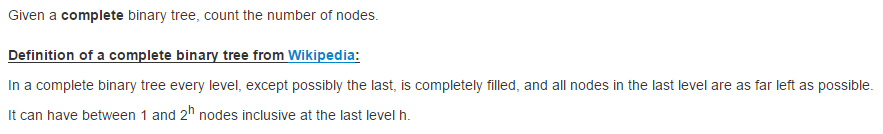分析
学过图论的我们知道，一棵层数为h的完整二叉树的最大节点数为2^h-1（节点的层数：从根开始定义起，根为第1层，根的子节点为第2层…）。那么问题来了，若结点范围是在2^(h-1)和2^h之间，怎么算呢？一种很直接的方法便是等于左子树的结点数+右子树的节点数+1。至此，我们就大概知道代码怎么写了。

如果刚好是满二叉树，那么节点数便是2^h-1;如果不是，那么就左子树、右子树的结点数分别加起来算，而左右子树结点的计算和刚刚的思想是一样的，所以递归就派上用场了。
代码
/**
* Definition for a binary tree node.
* struct TreeNode {
*     int val;
*     struct TreeNode *left;
*     struct TreeNode *right;
* };
*/
int countNodes(struct TreeNode* root) {
struct TreeNode *l=root,*r=root;
int hl=0,hr=0;

if(root==NULL)
return 0;

while(l){
l=l->left;
hl++;
}
while(r){
r=r->right;
hr++;
}

if(hl==1)
return 1;
else if(hl==hr)
return pow(2,hl)-1;
else
return countNodes(root->left)+countNodes(root->right)+1;
}

评价
上述方法是比较方便的一种方法。对于二叉树结点树的求解方法不止这种，我们还可以通过经典的遍历方法（前序遍历等）来统计数量，或者DFS来搜索完一次二叉树。

不考虑方法的高效和简洁的话，我们可以分别去统计树中度数为1、2、0的结点数进行累加。

展开全文c语言 遍历 递归
• 但是这里 问题就在于怎么计算点数出现的次数和。。一种是递归 一种是循环 递归 肯定效率不好。。 循环的话 想法很好。。 */ #include #include #include #include using namespace std; const int maxn=6; /
/*
题目是仍n个骰子 求各点数和出现的概率
概率计算么，一共是6^n 。。。然后计算各点数和出现的次数一除就行了么

但是这里 问题就在于怎么计算各点数出现的次数和。。一种是递归 一种是循环
递归 肯定效率不好。。
循环的话 想法很好。。
*/
#include<iostream>
#include<cstdio>
#include<cmath>
#include<cstring>
using namespace std;
const int maxn=6;
//递归的方法 n到十以上 性能就差的不行了。。
//还有就死和我递归函数写的确实不好。。 这么简单的递归也想不出来。
void Probability(int number,int cur,int sum,int * prob)
{
if(cur==1)
prob[sum-number]++;
else
{
for(int i=1;i<=maxn;++i)
Probability(number,cur-1,sum+i,prob);
}
}

void Probability(int number,int * prob)
{
for(int i=1;i<=maxn;++i)
Probability(number,number,i,prob);
}

void PrintProbability(int number)
{
if(number<1) return ;
int maxsum=maxn*number;
int * prob=new int[maxsum-number+1];
memset(prob,0,sizeof(prob));
Probability(number,prob);

int tot=pow((double)maxn,number);
for(int i=number;i<=maxsum;++i)
{
double ratio=(double)prob[i-number]/tot;
printf("%d %lf\n",i,ratio);
}
delete [] prob;

}
/*
下面开始写循环的，其实也有一点DP的思想
假如我们现在知道扔n-1个骰子 各点数出现的次数的数组了。。prob[i]就是i出现的次数
那么我们再扔一个骰子 也就其实是扔n个。 i点数出现的次数就是从i-1到i-6之间所有次数的和。
这样来回只需要两个数组 每次从一个数组推倒另一个数组 然后标记每次是该往哪个数组里存就可以了
这样性能就好的多了
*/
void PrintProbability_2(int num)
{
if(num<1) return ;
int * prob;
prob=new int[maxn*num+1];
prob=new int[maxn*num+1];
for(int i=1;i<=maxn*num;++i)
prob[i]=prob[i]=0;//这里有一个神奇bug 一开始忘记加i了 就是prob=0 0就是NULL这样就把指针清空了 所以后面错了
int flag=0;
for(int i=1;i<=maxn;++i)
prob[i]=1;
for(int i=2;i<=num;++i)
{
for(int j=0;j<i;++j)//因为扔i个骰子 最小合是i 所以i前面是0
prob[1-flag][j]=0;
for(int j=i;j<=maxn*i;++j)
{
prob[1-flag][j]=0;
for(int k=1;k<j && k<=maxn ;++k)
prob[1-flag][j]+=prob[flag][j-k];
}
flag=1-flag;
}

double tot=pow(double(maxn),num);
for(int i=num;i<=maxn*num;++i)
{
double ratio=(double)prob[flag][i]/tot;
printf("%d %lf\n",i,ratio);
}
delete[] prob;
delete[] prob;
}

int main()
{
int n;
while(cin>>n)
{
//PrintProbability(n);
cout<<endl;
PrintProbability_2(n);
}
return 0;
}

展开全文• 这里是转换为几何图形，利用点的分布计算出圆周率，画的不好看，明白怎么回事就行 以正方形的变长为1，圆形的半径为1，那么我知道知道第一象限圆的面积为π/4，然后正方形的面积为1，那么我们可以通过落入圆形中的...
这里是转换为几何图形，利用点的分布计算出圆周率，画的不好看，明白怎么回事就行

以正方形的变长为1，圆形的半径为1，那么我知道知道第一象限圆的面积为π/4，然后正方形的面积为1，那么我们可以通过落入圆形中的点数占的比例来估计π的值，顺便提一句，这个算法也叫蒙特卡洛概率算法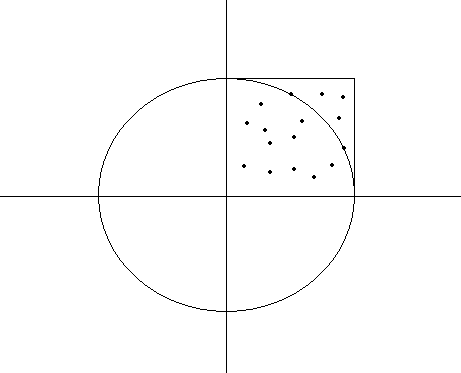以正方形的边长为1，圆形的半径为1，就知道第一象限圆的面积为π/4，然后正方形的面积为1，那么我们可以通过落入圆形中的点数占的比例来估计π的值，这个算法也叫蒙特卡洛概率算法

public class Test1 {
public static void main(String[] args) {
System.out.println("请输入取点数");
Scanner scanner = new Scanner(System.in);
int n = scanner.nextInt();
//调用方法进行计算
double pi = getPI(n);
System.out.println(pi);
}
public static double getPI(int n){
double pi = 0.0;
double x , y;
int res = 0;
for(int i = 0; i < n; i++){
x = Math.random();
y = Math.random();
//这里根据勾股定理进行计算，根据点到原点的距离判断该点是否在圆内，如果距离小于1，这说明在圆圈内，结果加1
if((x * x + y * y) < 1){
res++;
}
}
//这里必须写4.0     或者把4定义为double类型      否则4默认是int型   会发生数据类型转换
pi = 4.0 * res / n;
return pi;
}
}


展开全文• 题目：把N个骰子扔在地上，所有骰子朝上一面的点数之和为S，输入N，打印出S的所有可能的值出现的概率。 分析：对于6点的N个骰子来讲，其和S的...那么问题来了，怎么计算出S出现的次数呢？ 方案一：递归法。由于
题目：把N个骰子扔在地上，所有骰子朝上一面的点数之和为S，输入N，打印出S的所有可能的值出现的概率。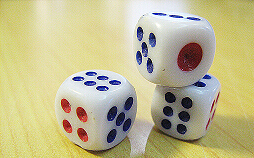分析：对于6点的N个骰子来讲，其和S的最小值为N，最大值为6N。要得到和S出现的概率，就得得到和S出现的次数，然后用某一S出现的次数/S的所有可能性=S出现的概率。对于骰子进行全排列，我们很容易知道S的所有可能性为：6^N。那么问题来了，怎么计算出S出现的次数呢？方案一：递归法。由于不能一口气吃一个大胖子，所以我们必须把事情一步步的解决。该方法的思路是将N个骰子分成两部分，第一部分是一个骰子，另一部分是剩下的N-1个。我们先计算第一部分第一个骰子出现的点数，很明显，有1-6这6种可能性；然后再计算这一已知的部分和剩下的N-1个骰子出现的点数之和。仿照前例，我们可以将第二部分分解为第一部分(1个)和第二部分（剩下的N-2）。我们把上一轮和这里的第一部分的和相加，得到新的点数之和，再将新的点数之和和剩下的N-2部分进行加法计算出新的点数之和。这就是典型的递归思想。结束条件是只剩下最后一个骰子。方案二：循环法。该方法的主要思路是用两个数组来求的骰子值可能出现的次数，是一种空间换时间的做法。关键思想是：在一次循环中，第一个数组中的第N个数字表示骰子和为N出现的次数。下一次循环中，我们加上一个新的骰子，此时和为S的骰子出现的次数应该等于第一个数组中S-1，S-2，S-3，S-4，S-5，S-6的次数之和。这样用一个数组来记录上一步的结果，而下一步的结果要用到上一步的结果。该方法避免了复杂多余的计算。
具体实现代码如下：#include <iostream>
#include <math.h>
#include <stdlib.h>
using namespace std;
int MaxValue=6;
void Probabilityhelp(int original,int current,int sum,int *probabilitys)
{
if(current==1)//当只剩下最后一个骰子的时候，计算其和；
{
probabilitys[sum-original]++;//和为sum的数组+1；
}
else
{
for(int i=1;i<=MaxValue;i++)
{
Probabilityhelp(original,current-1,sum+i,probabilitys);//剩下的继续递归；
}
}
}
void Probability(int number,int *pProbability)
{
for(int i=1;i<=MaxValue;i++)
Probabilityhelp(number,number,i,pProbability);
}
void PrintProbability(int number)
{
if(number<1)
return;
int MaxSum=number*MaxValue;
int *pProbability=new int[MaxSum-number+1];//我们定义一个6N-N+1的数组，和为S的点数出现的次数保存到数组第S-N个元素里。
for(int i=number;i<=MaxSum;i++)
pProbability[i-number]=0;//次数都初始化为0次；
Probability(number,pProbability);
int total=pow((double)MaxValue,number);//总共出现的值的可能性；
for(int i=number;i<=MaxSum;i++)
{
double ratio=(double)pProbability[i-number]/total;//出现的次数/总的可能性；
cout<<i<<" "<<ratio<<endl;
}
int a=1;
int b=2;
delete []pProbability;
}

int main()
{
PrintProbability(6);
system("pause");
return 0;
}
运行结果：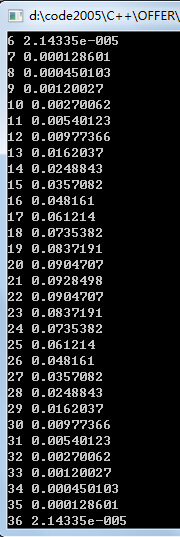由于该方法有许多地方的计算是重复的，和前面讲到过的【9】斐波拉切数列以及【39-1】中判断是否为平衡二叉树一样，存在重复计算导致效率低下。
所以下面采用一种循环的方式来解决此问题，也是时间换空间的方法。
方案二代码实现：#include <iostream>
#include <math.h>
using namespace std;
int g_maxValue=6;
void PrintProbability(int n)
{
if(n<1)
return;
int* pProbability;
pProbability=new int[g_maxValue*n+1];
pProbability=new int[g_maxValue*n+1];
for(int i=0;i<=g_maxValue*n;i++)
{
pProbability[i]=0;
pProbability[i]=0;
}
int flag=0;
for(int i=1;i<=g_maxValue;i++)
pProbability[flag][i]=1;
for(int k=2;k<=n;k++)
{
for(int i=0;i<k;i++)
pProbability[1-flag][i]=0;
for(int i=k;i<=g_maxValue*k;i++)
{
pProbability[1-flag][i]=0;
for(int j=1;j<=i && j<=g_maxValue;j++)
pProbability[1-flag][i]+=pProbability[flag][i-j];//这一步就是求和为S时的次数为上一步S-1，S-2，S-3，S-4，S-5，S-6的总次数；
}
flag=1-flag;
}
int total=pow((double)g_maxValue,n);
double prob=0;
for(int i=n;i<=g_maxValue*n;i++)
{
double ratio=(double)pProbability[flag][i]/total;
prob+=ratio;
cout<<i<<" "<<ratio<<" "<<endl;
}
cout<<"校验和是否1："<<prob<<endl;
cout<<endl;
delete[] pProbability;
delete[] pProbability;
}

int main()
{
PrintProbability(2);
system("pause");
return 0;
}
运行结果：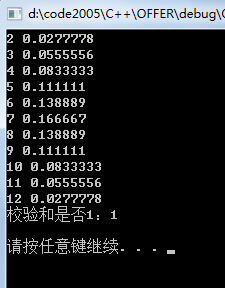此种方法的时间效率相对于方案有所提高，但是借助了辅助空间。

展开全文剑指offer
• 离散数学：一棵正则二叉树，叶子结点为T，求树的结点数？ 计算机网络：介质访问控制方式有哪些？ 操作系统：程序和进程的关系？ 机试：sizeof的作用，怎么通过sizeof求数组长度？ 政治：当代大学生如何理解德智...
• 下面的资料说实话 本人不怎么用 根据实际项目中的经验 有些公式计算所得和实际还是有差距的 一般本人还是用估算 水平子系统订购线缆计算实例 1、平均电缆长度=(最远F+最近N两条电缆总长)÷2 ...
• 1、首先来说数学期望怎么算 普遍解法的如下： 当我们要求的是从x个数值中随机挑选n个数值，且这x个数值为1->x的时候，这个时候求数学期望不需要按上面的解法，直接E(a)=n*(x+1)/2 2、伤害计算题目及解法 原题...字符串 算法
• 考虑怎么计算这种三元组，可以枚举s和f，然后计算从s到f的点不重复路径中可以经过的点的个数。 s到t点不重复路径中可以经过的点必定在也只能在这条路径所经过的点双内。所以可以考虑缩点双之后建出圆方树，然后就只...
• 这道题主要是要了解一个过程，就是动规转移方程是怎么构造的。这里面递推的时候要使用枚举的思想，假如有n条线，我们从一开始枚举，就是有一条直线平行，当然一条直线没法平行，意思就是，剩余的n-1条直线相交得到...
• 文章目录分段线性插值分段二次插值三次样条插值计算三次样条曲线s(x)三次样条插值的误差分析 多项式插值随着点数的增加，多项式的次数也在增加，由于龙格现象，所以多项式插值的误差也增加了。 那怎么做才可以使增加...
• 有毒。。。。 【题目分析】 首先讲一波错误的想法（来自...然后我们发现：哎呀这个点数怎么才2k啊，哇直接枚举所有对角线不就行了吗？ 然后我们再感性理解一下：在对角线两边，三角形的面积呈单峰函数。 所以就...
• 用一个叫做旋转卡壳的算法，我们可以在凸包顶点数的线性时间内求得最远点对 具体是怎么搞的呢？ 可以想象有两条平行线，“卡”住这个凸包，然后卡紧的情况下旋转一圈，肯定就能找 到凸包直径，也就找到了最...凸包
• 人机互动的出牌怎么出牌啊，求助各位大神，void cp()部分的代码 规则：一个人为用户，另一个为计算机，用户先出，轮流出牌，每次只能出一张并且要比别人大，如：用户出3，计算机要出比3大的数，没有则跳过，求出 ...c++ c语言
• 现给定A、B的各步跨出的格数，请计算比赛的结果。比赛结果只有4种情况：A胜，B胜，都未到达终点，A、B以相同的步数到达终点。 注意，长度为n的跑道上，格子的下标是从0开始，终点是下标为n-1的那个格子。先到达或...源代码
• 这个才是有意义的计算，否则计算出存储森林的二叉树的叶子结点数并没多大实用价值。OK,那么分析森林中的叶子结点在孩子结点的存储格式下的特点：左孩子指针域为空。该怎么理解这个？ 说到这里，需要特别强调的是...数据结构
• //功能：从主机向设备传入数组a、b，计算a、b数组的和，传入数组c中，再传回主机。 #include #include #include #include "device_launch_parameters.h" #define N 200000 __global__ void Add(int *d_a...cuda 优化 应用
• //i记录字符串，j记录加数节点数；s记录被加数节点数,w标记字符串中的'-'号, //k记录字符串中的字符转化为整数的值，l使每个节点记录4位 while(a[m]!=';') m++; //m记录字符串中被加数的字符数 n=m; while(a[n...数据 链表
• 其存储按IEEE754标准实现，即：1位的符号位s0（代表正数，1代表负数）8位的指数位e（移码表示）01111111是0的移码23位的小数位f0000000000000000000000表示小数部分为0计算机在表示符点数时，要将...
• //根据空白区域，计算各个字符的位图 List<Rectangle> charRects = new List(); for (int i = 1; i ; i++) { int diff = removeXs[i] - removeXs[i - 1]; if (diff > 5) { if (diff >= 20) { ...c#
• 被数到的最后一个人继续掷骰子，计算积分，依此类推。" 如果某人掷骰子后，其积分小于等于0则出列，余下的人继续按照此法进行游戏，直到剩下最后一个人，为胜利者。" ; } int main() { ...c++
• ## 投色子 游戏

千次阅读 2017-12-19 09:16:48
投色子游戏用Java怎么实现？ 下面菜鸡波就来教大家用Java实现一个不带窗体的投色子判胜游戏。 首先要制订游戏规则。我们这里采用人机对抗的形式。首先，人方投三次骰子，个得三个点数，然后，计算机投三次骰子得三...java
• 题意：坐标图上的多边形，...被题目误导了，在推出公式后一直在想怎么快速统计多边形内部点数。其实这道题目应该是逆着求的，先用叉积求多变形面积，再根据公式计算内部点数量。 #include using namespace std;struct
• 在地震勘探的科学计算中，我们经常用到三维数据体的读写，在MATLAB下怎么读取三维的数据体呢？其实很简单，代码如下：clc; close all; #定义一个空的三维数组 v2_res=zeros(187,801,801); #187：Z方向的点数 #801...MATLAB
•  题目的做法就是枚举两个点的组合，计算以这两个点为圆弧上两点时的圆心位置，然后根据这个圆心位置再遍历一遍所有点，计算处于圆内的点数，至于怎么计算圆心位置那就是几何问题了，具体可以看这里。时间复杂度为O...java python leetcode 算法 数据结构
• 1.先了解这几个关键字的概念 （1）分辨率。分辨率就是手机屏幕的像素点数，一般描述成屏幕的“宽×高”。720×1280表示此屏幕在宽度...2.知道手机尺寸和分辨率，怎么计算他的密度 假如我们知道一部手机的分辨率是...
• 1、在PS里选中文字的时候怎么查看文字的...2、行高怎么计算 line-height语法line-height属性的具体定义列表如下：语法： line-height : normal | <实数> | <长度> | <百分比> | inherit说明： ...